Comparison
Comparison close
Results

MEASURAND : Excess noise ratio
TRAVELLING STANDARD : Waveguide gas discharge noise generator
NOMINAL VALUE : 18 dB
FREQUENCY : 12.4 GHz

Degrees of equivalence: Di and expanded uncertainty Ui (k = 2), both expressed in dB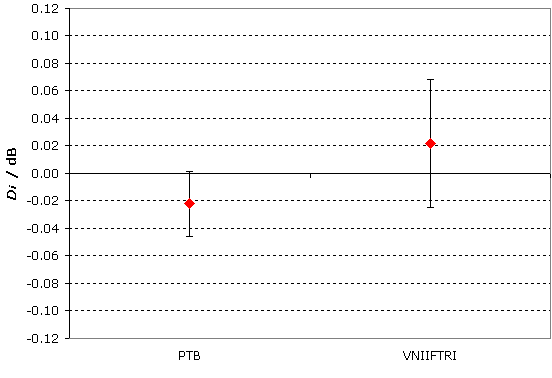MEASURAND : Excess noise ratio
TRAVELLING STANDARD : Waveguide gas discharge noise generator
NOMINAL VALUE : 18 dB
FREQUENCY : 13.5 GHz

Degrees of equivalence: Di and expanded uncertainty Ui (k = 2), both expressed in dB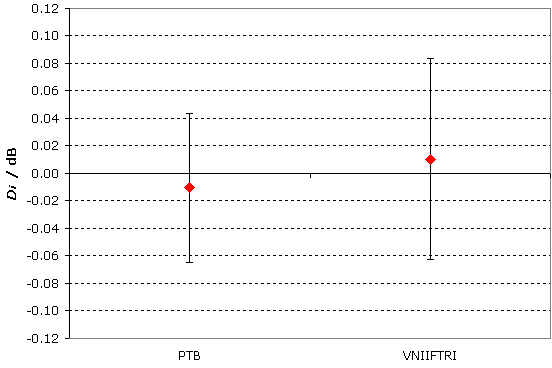MEASURAND : Excess noise ratio
TRAVELLING STANDARD : Waveguide gas discharge noise generator
NOMINAL VALUE : 18 dB
FREQUENCY : 15 GHz

Degrees of equivalence: Di and expanded uncertainty Ui (k = 2), both expressed in dB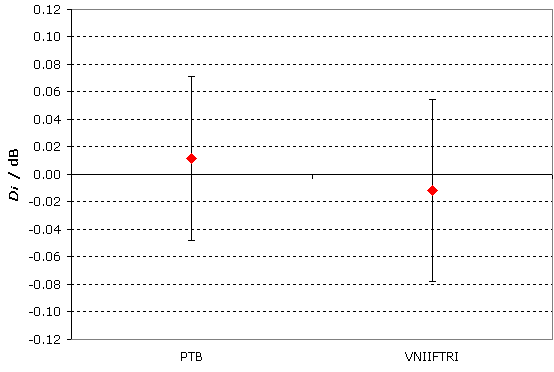MEASURAND : Excess noise ratio
TRAVELLING STANDARD : Waveguide gas discharge noise generator
NOMINAL VALUE : 18 dB
FREQUENCY : 17.5 GHz

Degrees of equivalence: Di and expanded uncertainty Ui (k = 2), both expressed in dB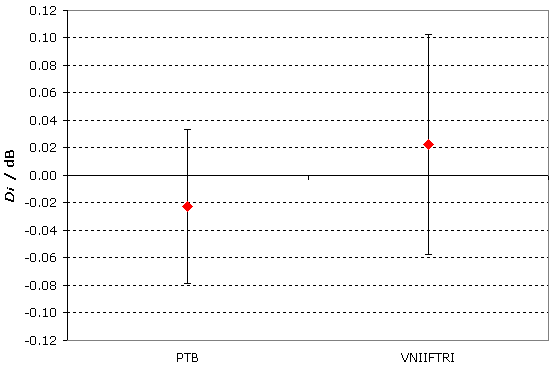MEASURAND : Noise temperature
TRAVELLING STANDARD : Waveguide gas discharge noise generator
FREQUENCY : 12.4 GHz

Degrees of equivalence: Di and expanded uncertainty Ui (k = 2), both expressed in K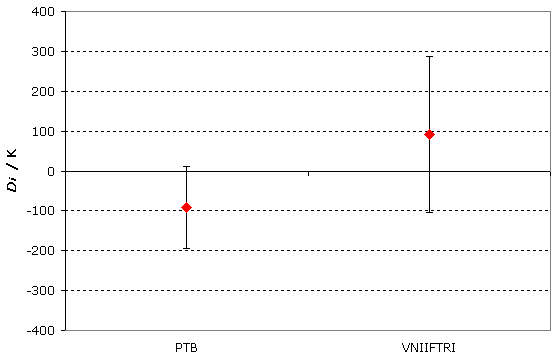MEASURAND : Noise temperature
TRAVELLING STANDARD : Waveguide gas discharge noise generator
FREQUENCY : 13.5 GHz

Degrees of equivalence: Di and expanded uncertainty Ui (k = 2), both expressed in K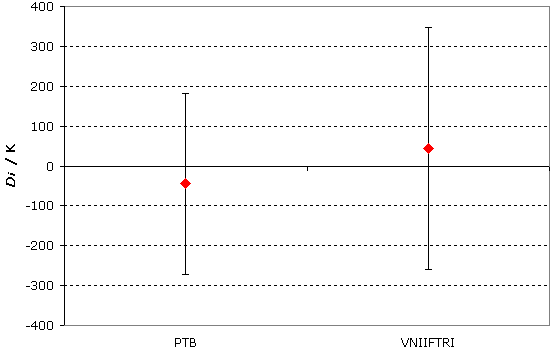MEASURAND : Noise temperature
TRAVELLING STANDARD : Waveguide gas discharge noise generator
FREQUENCY : 15 GHz

Degrees of equivalence: Di and expanded uncertainty Ui (k = 2), both expressed in K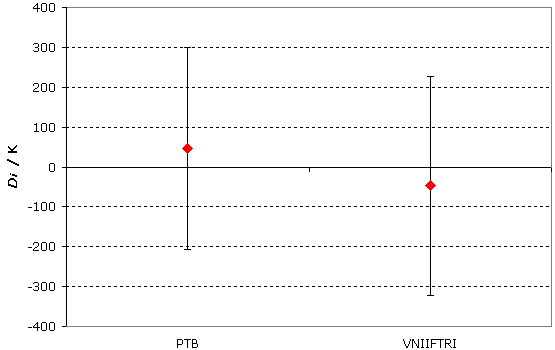MEASURAND : Noise temperature
TRAVELLING STANDARD : Waveguide gas discharge noise generator
FREQUENCY : 17.5 GHz

Degrees of equivalence: Di and expanded uncertainty Ui (k = 2), both expressed in K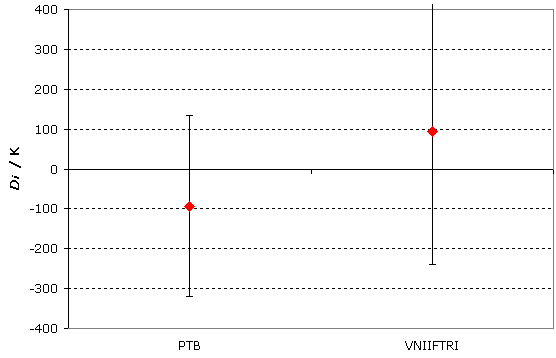Comparison
Comparison close
Results

MEASURAND : Excess noise ratio
TRAVELLING STANDARD : Waveguide gas discharge noise generator
NOMINAL VALUE : 18 dB
FREQUENCY : 12.4 GHz

Degrees of equivalence: Di and expanded uncertainty Ui (k = 2), both expressed in dB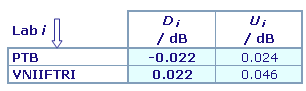Results are presented under A4 printable format in Summary Results (.PDF file).

MEASURAND : Excess noise ratio
TRAVELLING STANDARD : Waveguide gas discharge noise generator
NOMINAL VALUE : 18 dB
FREQUENCY : 13.5 GHz

Degrees of equivalence: Di and expanded uncertainty Ui (k = 2), both expressed in dB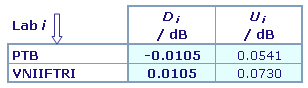Results are presented under A4 printable format in Summary Results (.PDF file).

MEASURAND : Excess noise ratio
TRAVELLING STANDARD : Waveguide gas discharge noise generator
NOMINAL VALUE : 18 dB
FREQUENCY : 15 GHz

Degrees of equivalence: Di and expanded uncertainty Ui (k = 2), both expressed in dB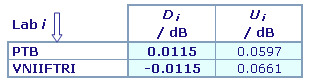Results are presented under A4 printable format in Summary Results (.PDF file).

MEASURAND : Excess noise ratio
TRAVELLING STANDARD : Waveguide gas discharge noise generator
NOMINAL VALUE : 18 dB
FREQUENCY : 17.5 GHz

Degrees of equivalence: Di and expanded uncertainty Ui (k = 2), both expressed in dB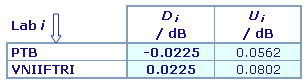Results are presented under A4 printable format in Summary Results (.PDF file).

MEASURAND : Noise temperature
TRAVELLING STANDARD : Waveguide gas discharge noise generator
FREQUENCY : 12.4 GHz

Degrees of equivalence: Di and expanded uncertainty Ui (k = 2), both expressed in K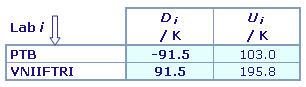Results are presented under A4 printable format in Summary Results (.PDF file).

MEASURAND : Noise temperature
TRAVELLING STANDARD : Waveguide gas discharge noise generator
FREQUENCY : 13.5 GHz

Degrees of equivalence: Di and expanded uncertainty Ui (k = 2), both expressed in K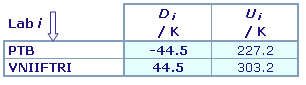Results are presented under A4 printable format in Summary Results (.PDF file).

MEASURAND : Noise temperature
TRAVELLING STANDARD : Waveguide gas discharge noise generator
FREQUENCY : 15 GHz

Degrees of equivalence: Di and expanded uncertainty Ui (k = 2), both expressed in K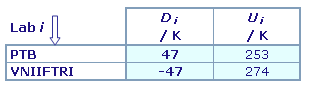Results are presented under A4 printable format in Summary Results (.PDF file).

MEASURAND : Noise temperature
TRAVELLING STANDARD : Waveguide gas discharge noise generator
FREQUENCY : 17.5 GHz

Degrees of equivalence: Di and expanded uncertainty Ui (k = 2), both expressed in K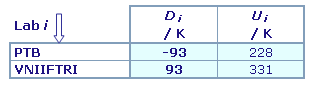Results are presented under A4 printable format in Summary Results (.PDF file).

Comparison
Comparison close
CC comparison
 Metrology area, Sub-field Electricity and Magnetism, Radio frequencies Description Excess noise ratio / noise temperature Time of measurements 2003 - 2007 Status Approved for equivalence
 Final Reports of the comparisons Measurand Noise temperature and excess noise ratio Parameters Frequency: 12.4 GHz, 13.5 GHz, 15.0 GHz and 17.5 GHz Transfer device Waveguide gas discharge noise generator, power supply and reserve gas discharge tube
 Comparison type Key Comparison Consultative Committee CCEM (Consultative Committee for Electricity and Magnetism) Conducted by CCEM (Consultative Committee for Electricity and Magnetism) Comments This comparison is a bilateral key comparison subsequent to CCEM.RF-K9. It was undertaken to provide VNIIFTRI an opportunity to confirm that it had corrected problems that it experienced in CCEM.RF-K9. Compared to CCEM.RF-K9, CCEM.RF-K9.1 used fewer travelling standards, did not address the effects of adapters, and used a travelling standard with a substantially different ENR (approximately 18 dB instead of approximately 16 dB or 4 dB, as in CCEM.RF-K9). Therefore, it was decided that there would be no formal linkage of CCEM.RF-K9.1 results to those of CCEM.RF-K9.Results published on 29 July 2009 Noise temperature and excess noise ratio Pilot institute LNE Laboratoire national de métrologie et d'essais France Contact person D. Allal +33 (0) 1 30 69 21 50
First Name Last Name
wwww@ww.www +356719836 Institute 1 Institute 1 Khmelnitskiy
Pilot laboratory
LNE

Laboratoire national de métrologie et d'essais, France, EURAMET

 PTB Physikalisch-Technische Bundesanstalt, Germany, EURAMET
 VNIIFTRI Institute of Physical Technical and Radiotechnical Measurements, Rosstandart, Russian Federation, COOMET
Comparison
Comparison close
Results

MEASURAND : Excess noise ratio
TRAVELLING STANDARD : Waveguide gas discharge noise generator
NOMINAL VALUE : 18 dB
FREQUENCY : 12.4 GHz

 The key comparison reference value xENR R is calculated as the unweighted mean of the participants results. Its standard uncertainty uENR R is calculated as the experimental standard deviation of the mean. xENR R = 17.926 dB, uENR R = 0.022 dB

 The degree of equivalence of laboratory i with respect to the key comparison reference value is given by two terms both expressed in dB: Di = (xENR i - xENR R) and its expanded uncertainty Ui (k = 2).

 No pair-wise degrees of equivalence are computed for this comparison.

MEASURAND : Excess noise ratio
TRAVELLING STANDARD : Waveguide gas discharge noise generator
NOMINAL VALUE : 18 dB
FREQUENCY : 13.5 GHz

 The key comparison reference value xENR R is calculated as the unweighted mean of the participants results. Its standard uncertainty uENR R is calculated as the experimental standard deviation of the mean. xENR R = 17.9415 dB, uENR R = 0.0105 dB

 The degree of equivalence of laboratory i with respect to the key comparison reference value is given by two terms both expressed in dB: Di = (xENR i - xENR R) and its expanded uncertainty Ui (k = 2).

 No pair-wise degrees of equivalence are computed for this comparison.

MEASURAND : Excess noise ratio
TRAVELLING STANDARD : Waveguide gas discharge noise generator
NOMINAL VALUE : 18 dB
FREQUENCY : 15 GHz

 The key comparison reference value xENR R is calculated as the unweighted mean of the participants results. Its standard uncertainty uENR R is calculated as the experimental standard deviation of the mean. xENR R = 17.9175 dB, uENR R = 0.0115 dB

 The degree of equivalence of laboratory i with respect to the key comparison reference value is given by two terms both expressed in dB: Di = (xENR i - xENR R) and its expanded uncertainty Ui (k = 2).

 No pair-wise degrees of equivalence are computed for this comparison.

MEASURAND : Excess noise ratio
TRAVELLING STANDARD : Waveguide gas discharge noise generator
NOMINAL VALUE : 18 dB
FREQUENCY : 17.5 GHz

 The key comparison reference value xENR R is calculated as the unweighted mean of the participants results. Its standard uncertainty uENR R is calculated as the experimental standard deviation of the mean. xENR R = 17.9275 dB, uENR R = 0.0225 dB

 The degree of equivalence of laboratory i with respect to the key comparison reference value is given by two terms both expressed in dB: Di = (xENR i - xENR R) and its expanded uncertainty Ui (k = 2).

 No pair-wise degrees of equivalence are computed for this comparison.

MEASURAND : Noise temperature
TRAVELLING STANDARD : Waveguide gas discharge noise generator
FREQUENCY : 12.4 GHz

 The key comparison reference value xT R is calculated as the unweighted mean of the participants results. Its standard uncertainty uT R is calculated as the experimental standard deviation of the mean. xT R = 18278.5 K, uT R = 91.5 K

 The degree of equivalence of laboratory i with respect to the key comparison reference value is given by two terms both expressed in K: Di = (xT i - xT R) and its expanded uncertainty Ui (k = 2).

 No pair-wise degrees of equivalence are computed for this comparison.

MEASURAND : Noise temperature
TRAVELLING STANDARD : Waveguide gas discharge noise generator
FREQUENCY : 13.5 GHz

 The key comparison reference value xT R is calculated as the unweighted mean of the participants results. Its standard uncertainty uT R is calculated as the experimental standard deviation of the mean. xT R = 18342.5 K, uT R = 44.5 K

 The degree of equivalence of laboratory i with respect to the key comparison reference value is given by two terms both expressed in K: Di = (xT i - xT R) and its expanded uncertainty Ui (k = 2).

 No pair-wise degrees of equivalence are computed for this comparison.

MEASURAND : Noise temperature
TRAVELLING STANDARD : Waveguide gas discharge noise generator
FREQUENCY : 15 GHz

 The key comparison reference value xT R is calculated as the unweighted mean of the participants results. Its standard uncertainty uT R is calculated as the experimental standard deviation of the mean. xT R = 18243 K, uT R = 47 K

 The degree of equivalence of laboratory i with respect to the key comparison reference value is given by two terms both expressed in K: Di = (xT i - xT R) and its expanded uncertainty Ui (k = 2).

 No pair-wise degrees of equivalence are computed for this comparison.

MEASURAND : Noise temperature
TRAVELLING STANDARD : Waveguide gas discharge noise generator
FREQUENCY : 17.5 GHz

 The key comparison reference value xT R is calculated as the unweighted mean of the participants results. Its standard uncertainty uT R is calculated as the experimental standard deviation of the mean. xT R = 18285 K, uT R = 93 K

 The degree of equivalence of laboratory i with respect to the key comparison reference value is given by two terms both expressed in K: Di = (xT i - xT R) and its expanded uncertainty Ui (k = 2).

 No pair-wise degrees of equivalence are computed for this comparison.
Comparison
Comparison close
Results

MEASURAND : Excess noise ratio
TRAVELLING STANDARD : Waveguide gas discharge noise generator
NOMINAL VALUE : 18 dB
FREQUENCY : 12.4 GHz

xENR i : Excess noise ratio measured by laboratory i
uENR i : standard uncertainty of xENR i

 Lab i xENR i / dB uENR i / dB Date of measurement PTB 17.904 0.025 Dec 03 VNIIFTRI 17.948 0.032 Feb - Apr 07

MEASURAND : Excess noise ratio
TRAVELLING STANDARD : Waveguide gas discharge noise generator
NOMINAL VALUE : 18 dB
FREQUENCY : 13.5 GHz

xENR i : Excess noise ratio measured by laboratory i
uENR i : standard uncertainty of xENR i

 Lab i xENR i / dB uENR i / dB Date of measurement PTB 17.931 0.029 Dec 03 VNIIFTRI 17.952 0.038 Feb - Apr 07

MEASURAND : Excess noise ratio
TRAVELLING STANDARD : Waveguide gas discharge noise generator
NOMINAL VALUE : 18 dB
FREQUENCY : 15 GHz

xENR i : Excess noise ratio measured by laboratory i
uENR i : standard uncertainty of xENR i

 Lab i xENR i / dB uENR i / dB Date of measurement PTB 17.929 0.032 Dec 03 VNIIFTRI 17.906 0.035 Feb - Apr 07

MEASURAND : Excess noise ratio
TRAVELLING STANDARD : Waveguide gas discharge noise generator
NOMINAL VALUE : 18 dB
FREQUENCY : 17.5 GHz

xENR i : Excess noise ratio measured by laboratory i
uENR i : standard uncertainty of xENR i

 Lab i xENR i / dB uENR i / dB Date of measurement PTB 17.905 0.036 Dec 03 VNIIFTRI 17.950 0.046 Feb - Apr 07

MEASURAND : Noise temperature
TRAVELLING STANDARD : Waveguide gas discharge noise generator
FREQUENCY : 12.4 GHz

xT i : Noise temperature measured by laboratory i
uT i : standard uncertainty of xT i

 Lab i xT i / K uT i / K Date of measurement PTB 18187 105 Dec 03 VNIIFTRI 18370 134 Feb - Apr 07

MEASURAND : Noise temperature
TRAVELLING STANDARD : Waveguide gas discharge noise generator
FREQUENCY : 13.5 GHz

xT i : Noise temperature measured by laboratory i
uT i : standard uncertainty of xT i

 Lab i xT i / K uT i / K Date of measurement PTB 18298 122 Dec 03 VNIIFTRI 18387 158 Feb - Apr 07

MEASURAND : Noise temperature
TRAVELLING STANDARD : Waveguide gas discharge noise generator
FREQUENCY : 15 GHz

xT i : Noise temperature measured by laboratory i
uT i : standard uncertainty of xT i

 Lab i xT i / K uT i / K Date of measurement PTB 18290 135 Dec 03 VNIIFTRI 18196 145 Feb - Apr 07

MEASURAND : Noise temperature
TRAVELLING STANDARD : Waveguide gas discharge noise generator
FREQUENCY : 17.5 GHz

xT i : Noise temperature measured by laboratory i
uT i : standard uncertainty of xT i

 Lab i xT i / K uT i / K Date of measurement PTB 18192 147 Dec 03 VNIIFTRI 18378 190 Feb - Apr 07